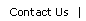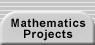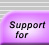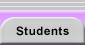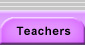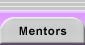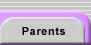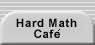# Fermat's Little Theorem

Fermat's little theorem states that for an integer, n, and a prime, p, that does not divide n,

np-1 = 1 (mod p).

For example, for p = 5,

24 = 16 and 16 = 1 (mod 5)

34 = 81 and 81 = 1 (mod 5)

44 = 256 and 256 = 1 (mod 5)

64 = 1296 and 1296 = 1 (mod 5)

etc.

See Fermat's Little Theorem and its links (especially its interactive modular arithmetic table) for an introduction and proof. Notation note: a|p means that p divides a.

For a more advanced discussion and additional proofs, see How to Discover the Statement and Two Proofs of Fermat's Little Theorem.Translations of mathematical formulas for web display were created by tex4ht. © Copyright 2003 Education Development Center, Inc. (EDC)